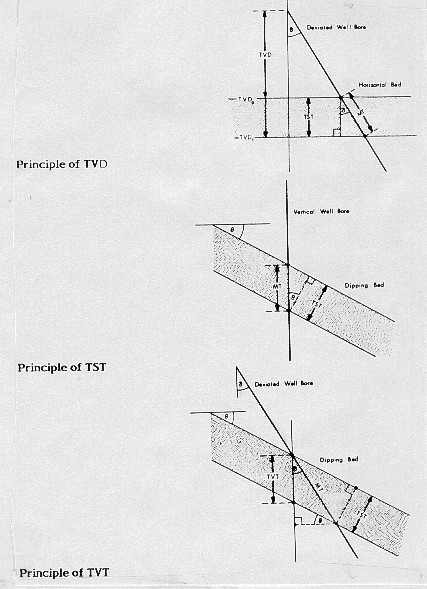True Stratigraphic / True Vertical Thickness
True stratigraphic and true vertical thickness are important in dipping beds and in deviated holes, since reservoir volume depends on these properties and not the measured thickness. The formulas are documented in "The Log Analyst and the Programmable Calculator" by R. Bateman and C. Konen in The Log Analyst, Mar 1979.

Definitions of the terms are illustrated below.Geometry for TVD, TVT, and TST calculations

1: TST = MT * (Cos WD * Cos DIP - Sin WD * Sin DIP * Cos (HAZ - AZM))
2: TVT = TST / Cos DIP

Where:
AZM = true dip azimuth
DIP = true dip angle
HAZ = azimuth of hole direction relative to true north
MT = measured thickness (feet or meters)
TST = true stratigraphic thickness (feet or meters)
TVT = true vertical thickness (feet or meters)
WD = well deviation angle

Page Views ---- Since 01 Jan 2015